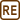## Efficiency of Carnot Engine

### Task number: 1802

Carnot engine operates with efficiency of 40 %. How much must the temperature of the hot reservoir increase, so that the efficiency increases to 50 %? The temperature of the cold reservoir remains at 9 °C.

• #### Hint

Carnot efficiency is given by the relation

$\eta=\frac{T_1-T_2}{T_1},$

where T1 is the temperature of hot reservoir and T2 is the temperature of cold reservoir.

• #### Analysis

Write down the relationships for the initial efficiency of the Carnot engine and for the efficiency after changing the temperature of the hot reservoir. Using these equations, evaluate the initial and the final temperature of the hot reservoir. The temperature increase of the hot reservoir can be determined as the difference of these two temperatures.

• #### Given values

 η1 = 40 % = 0.4 initial efficiency of Carnot engine η2 = 50 % = 0.5 efficiency of Carnot engine after increasing the temperature of hot reservoir t2 = 9 °C => T2 = 282 K temperature of cold reservoir ΔT1 = ? temperature increase of hot reservoir
• #### Solution

First we write down the relationships for the initial efficiency η1 of Carnot engine and for the efficiency η2 after changing the temperature of the hot reservoir:

$\eta_1=\frac{T_1-T_2}{T_1}, \qquad \qquad \qquad \eta_2=\frac{T_1^*-T_2}{T_1^*},$

where T1 is the initial temperature of the hot reservoir, T1* is the new temperature of the hot reservoir, and T2 is the temperature of the cold reservoir.

Now we evaluate the unknown temperatures T1 and T1* from these relations:

$T_1=\frac{T_2}{1-\eta_1}, \qquad \qquad \qquad T_1^*=\frac{T_2}{1-\eta_2}.$

The unknown temperature difference ΔT1 of the hot reservoir is given by:

$\Delta T_1=T_1^*-T_1$ and by substituting the above evaluated temperatures we obtain $\Delta T_1=\frac{T_2}{1-\eta_2}-\frac{T_2}{1-\eta_1}.$
• #### Numerical solution

$\Delta T_1=\frac{T_2}{1-\eta_2}-\frac{T_2}{1-\eta_1}=\left(\frac{282}{1-0.5}-\frac{282}{1-0.4}\right)\,\mathrm{K}\,\dot{=}\, 94\,\mathrm{K}$
• #### Answer

The temperature of the hot reservoir must increase by 94 K, i.e. by 94 °C.